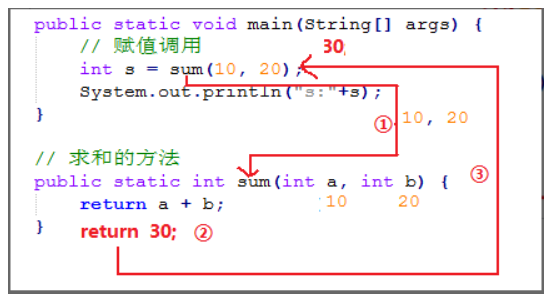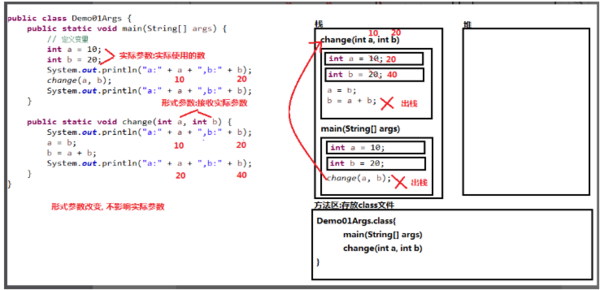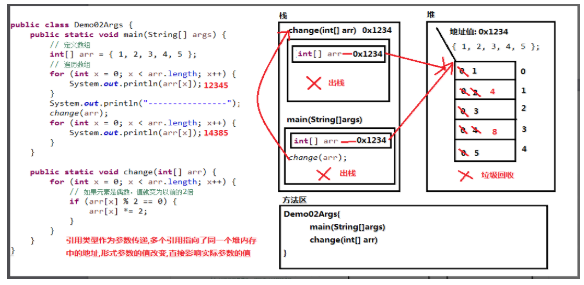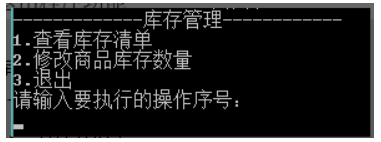编程技术资讯
 程序员段子
 程序人生
 秒杀专栏
 名企资讯
 运维监控
 悟空教程-Java
 悟空教程-Python
 悟空教程-大数据
 人事面试
 技术面试
 大禹编程
 SpringBoot
 SpringCloud(F版)
 SpringCloud(D版)
 spring
 Docker
 Dubbo
 IntelliJ IDEA
 Linux
 Nginx
 Tomcat
 Quartz
 微信小程序
 阿里云
 Java
 Python
 Java案例
 小程序源码
 Java源码
 手机最新资讯
 Sql Server
 MySQL
 MongoDB
 免费教程
 前端开发
 node.js
 CSS
 移动端
 jQuery
 Sublime Test
 JavaScript
 HTML
 Vue.js
 HTTPS
 分布式事物
 Redis
 JSON
 DevOps

# 第五天 方法【悟空教程】

10805天 方法

# 第1章 方法

## 1.1 方法概述

main方法就是一个特殊的方法。作为程序入口，供JVM调用。

## 1.2 方法的定义及使用

### 1.2.1 方法定义格式

………

return 返回值;

}

• 修饰符：方法的修饰符比较多，有对访问权限进行限定的，有静态修饰符static，还有最终修饰符final等，这些修饰符在后面的学习过程中会逐步介绍。目前使用固定的public static修饰符

• 返回值类型：方法返回值的数据类型

• 参数类型：调用方法时传入参数的数据类型

• 参数名：是一个变量，用于接收调用方法时传入的数据

• return关键字：用于结束方法以及返回返回值

• 返回值：被return语句返回的值，该值会返回给调用者

### 1.2.2 方法的使用格式

#### 1.2.2.1 方法的使用：

/*

………

return 返回值;

}

return关键字：用于结束方法以及返回返回值

*/

public class Demo01Method{

public static void main(String[] args){

//调用方法

System.out.println("c  = " + c);

}

//定义求两个整数和方法    3     4

public static int add(int a,int b){

System.out.println("求两个整数的和方法开始");

int sum = a + b;

System.out.println("求两个整数的和方法结束");

return sum;//7

}

}

#### 1.2.2.2 调用方法介绍：求和方法编写

public class Demo01Method {

/*

* 写一个方法，用于求和。

* 两个明确： 返回值类型 int 参数列表int a,int b

*/

public static int sum(int a,int b){

/*int c = a + b;

return c;*/

return a + b;

}

}

• 求和方法的调用(有明确返回值)

• 使用格式：方法名(参数);

• 单独调用，没有意义

sum(10,20);

• 输出调用，有意义，但是不够好，因为我不一定非要把结果输出

System.out.println(sum(10,20));

• 赋值调用，推荐方式

int s = sum(10, 20);

// s+=100;

System.out.println("s:"+s);

• 求和方法的调用图解### 1.2.3 定义格式注意事项

a) 返回值类型

b) return

c) 返回值

d) 方法名

e) 形式参数

/*

a)返回值类型

b)return

c)返回值

d)方法名

e)形式参数

*/

public class Demo02Method{

public static void main(String[] args){

//调用唐僧生孩子方法

//String xingMing = shengHaiZi(36);

//System.out.println("名字是: " + xingMing);

//唐僧生一群孩子方法

String[] xingMings = shengYiQunHaiZi(108);

for(int i=0; i<xingMings.length; i++){

System.out.println(""+(i+1)+"孩子的姓名是: " +xingMings[i]);

}

//坐月子的方法

zuoYueZi(1800);

}

//定义一个唐僧生孩子方法

public static String shengHaiZi(int month){

System.out.println("怀了"+month+"个月,终于生孩子了!");

String name = "糖三角";

return name;

}

//定义一个唐僧生一群孩子方法

public static String[] shengYiQunHaiZi(int month){

System.out.println("怀了"+month+"个月,终于生了一群孩子了!");

String[] names = {"孙悟空","猪八戒","沙师弟","白龙马"};

return names;

}

//定义一个坐月子的方法

public static void zuoYueZi(int day){

System.out.println("在床上躺了"+day+"!");

//return;

}

}

### 1.2.4 定义格式void与参数详解：

#### 1.2.4.1 Void修饰的方法

• 格式

return 返回值;

}

• 没有明确返回值的函数调用:

• 其实就是void类型方法的调用

• 只能单独调用

• 案例

public class Demo01Method {

public static void main(String[] args) {

//单独调用

printHelloWorld();

}

//在控制台输出10HelloWorld案例。

public static void printHelloWorld() {

for(int x=1; x<=10; x++) {

System.out.println("HelloWorld");

}

}

}

#### 1.2.4.2 Void修饰方法练习

##### 1.2.4.2.1 定义一个方法，传递一个整数(大于1)，在控制台打印1到该数据的值。

public class Demo02Method {

public static void main(String[] args) {

printNumber(10);

System.out.println("-------------------");

printNumber(100);

}

//在控制台打印1到该数据n的值

public static void printNumber(int n) {

for(int x=1; x<=n; x++) {

System.out.println(x);

}

}

}

##### 1.2.4.2.2定义一个方法，把所有的水仙花数打印在控制台

public class Demo03Method {

public static void main(String[] args) {

printFlower();

}

//把所有的水仙花数打印在控制台

public static void printFlower() {

for(int x=100; x<1000; x++) {

int ge = x%10;

int shi = x/10%10;

int bai = x/10/10%10;

if((ge*ge*ge+shi*shi*shi+bai*bai*bai) == x){

System.out.println(x);

}

}

}

}

### 1.2.5 方法参数传递问题

#### 1.2.5.1 参数传递概述

• 在这里，定义方法时，参数列表中的变量，我们称为形式参数

• 调用方法时，传入给方法的数值，我们称为实际参数

#### 1.2.5.2 参数是基本类型及图解

n 形式参数的改变不影响实际参数。

public class Demo01Args {

public static void main(String[] args) {

// 定义变量

int a = 10;

int b = 20;

System.out.println("a:" + a + ",b:" + b);// a:10,b:20

change(a, b);

System.out.println("a:" + a + ",b:" + b);// a:10,b:20

}

public static void change(int a, int b) { // a=10,b=20

System.out.println("a:" + a + ",b:" + b);// a:10,b:20

a = b; // a=20;

b = a + b;// b=40;

System.out.println("a:" + a + ",b:" + b);// a:20,b:40

}

}#### 1.2.5.3 参数是引用类型及图解

public class Demo02Args {

public static void main(String[] args) {

// 定义数组

int[] arr = { 1, 2, 3, 4, 5 };

// 遍历数组

for (int x = 0; x < arr.length; x++) {

System.out.println(arr[x]);

}

System.out.println("----------------");

change(arr);

for (int x = 0; x < arr.length; x++) {

System.out.println(arr[x]);

}

}

public static void change(int[] arr) {

for (int x = 0; x < arr.length; x++) {

// 如果元素是偶数，值就变为以前的2

if (arr[x] % 2 == 0) {

arr[x] *= 2;

}

}

}

}### 1.2.6 多个方法可以共同访问成员变量

/*

*/

public class Demo03Method{

//int x;//错误: 无法从静态上下文中引用非静态 变量 x

static int x;

public static void main(String[] args){

x = 100;

System.out.println("x = " + x);

//调用method2方法

method2();

}

//方法1

public static int method1(int a){

int b = 10;

System.out.println(a*b);

return a*b;

}

//方法2

public static void method2(){

//System.out.println(a*b);// 错误: 找不到符号 方法2不能使用方法1里边的变量

//x = 200;

System.out.println("x = " + x);

}

}

## 1.3 方法定义练习

### 1.3.4 有返回值有参数方法，如求三个数的平均值

/*

*/

import java.util.Scanner;

public class Demo04Method{

public static void main(String[] args){

//调用打印矩形方法

//printJX();

//调用打印不同矩形方法

//printJuXing(9,9);

//printJuXing(7,6);

//调用获取整数的方法

int a = getNumber();

System.out.println("a = " + a);

//调用求3个小数平均数方法

double b = pingJun(3.0,4.0,5.0);

System.out.println("b = " + b);

}

/*

*/

public static void printJX(){

/*

***

***

***

*/

for(int i=0; i<3; i++){//控制打印行数

for(int j=0; j<3; j++){//控制每行打印几个

System.out.print("*");

}

System.out.println();

}

}

/*

*/

public static void printJuXing(int m, int n){

for(int i=0; i<m; i++){//控制打印行数

for(int j=0; j<n; j++){//控制每行打印几个

System.out.print("*");

}

System.out.println();

}

}

/*

*/

public static int getNumber(){

Scanner sc = new Scanner(System.in);

System.out.println("请输入一个整数: ");

int number = sc.nextInt();

return number;

}

/*

*/

public static double pingJun(double a,double b,double c){

//double avg = (a + b + c)/3;

return (a + b + c)/3;

}

}

### 1.3.5 获取两个数据中的较大值

public class Demo03MethodTest {

public static void main(String[] args) {

//创建对象

Scanner sc = new Scanner(System.in);

//接收数据

System.out.println("请输入第一个数据：");

int x = sc.nextInt();

System.out.println("请输入第二个数据：");

int y = sc.nextInt();

//调用方法

int max = getMax(x,y);

System.out.println("max:"+max);

}

// 返回两个数中的较大值

public static int getMax(int a, int b) {

if (a > b) {

return a;

} else {

return b;

}

}

}

### 1.3.6 比较两个数据是否相等

public class Demo04MethodTest {

public static void main(String[] args) {

//创建对象

Scanner sc = new Scanner(System.in);

//接收数据

System.out.println("请输入第一个数据:");

int a = sc.nextInt();

System.out.println("请输入第二个数据:");

int b = sc.nextInt();

//调用方法

boolean flag = compare(a,b);

System.out.println("flag:"+flag);

}

//比较两个数是否相等

public static boolean compare(int a,int b){

if(a==b){

return true;

}else {

return false;

}

}

}

### 1.3.7 获取三个数据中的较大值

public class Demo05MethodTest {

public static void main(String[] args) {

//创建对象

Scanner sc = new Scanner(System.in);

//接收数据

System.out.println("请输入第一个数据：");

int a = sc.nextInt();

System.out.println("请输入第二个数据：");

int b = sc.nextInt();

System.out.println("请输入第三个数据：");

int c = sc.nextInt();

//调用方法

int max = getMax(a,b,c);

System.out.println("max:"+max);

}

// 返回三个数中的最大值

public static int getMax(int a, int b, int c) {

if (a > b) {

if (a > c) {

return a;

} else {

return c;

}

} else {

if (b > c) {

return b;

} else {

return c;

}

}

}

}

## 1.4 方法重载

### 1.4.1 方法重载定义

public static int add(int a,int b) {逻辑} //两个数加法

public static int add(int a,int b,int c) {逻辑} //三个数加法

public static int add(int a,int b,int c,int d) {逻辑} //四个数加法

/*

*/

public static void main(String[] args){

}

//两个数加法

public static int add(int a,int b) {

System.out.println("调用两个数相加");

return a + b;

}

//三个数加法

public static int add(int a,int b,int c) {

System.out.println("调用三个数相加");

return a + b + c;

}

//四个数加法

public static int add(int a,int b,int c,int d) {

System.out.println("调用四个数相加");

return a + b + c + d;

}

}

### 1.4.2 重载的注意事项

a) 重载方法参数必须不同：

b) 重载只与方法名与参数类型相关与返回值无关

c) 重载与具体的变量标识符无关

/*

a)重载方法参数必须不同：

b)重载只与方法名与参数类型相关与返回值无关

void method(int x)int method(int y)不是方法重载，不能同时存在

c)重载与具体的变量标识符无关

method(int x)method(int y)不是方法重载，不能同时存在

*/

public static void main(String[] args){

//求两个小数的和

//System.out.println("sum = " + sum);

//求两个整数的和

}

//两个数加法

public static int add(int a,double b) {

System.out.println("调用先int两个数相加");

return (int)(a + b);

}

//两个数加法

/*

c)重载与具体的变量标识符无关

method(int x)method(int y)不是方法重载，不能同时存在

public static int add(int x,double y) {

System.out.println("调用先int两个数相加");

return (int)(x + y);

}

*/

//两个数加法

public static double add1(int a,double b) {

System.out.println("调用先int两个数相加");

return (a + b);

}

//两个数加法

public static double add(double a,int b) {

System.out.println("调用先double两个数相加");

return a + b;

}

//两个数小数加法

public static double add(double a,double b) {

System.out.println("调用两个小数相加");

return a + b;

}

//三个数加法

public static int add(int a,int b,int c) {

System.out.println("调用三个数相加");

return a + b + c;

}

//四个数加法

public static int add(int a,int b,int c,int d) {

System.out.println("调用四个数相加");

return a + b + c + d;

}

}

### 1.4.3 练习

#### 1.4.3.1 比较两个数据是否相等。

public class Demo02MethodTest {

public static void main(String[] args) {

// 调用

System.out.println(compare(10, 20));//整数默认为int类型

System.out.println("-------------");

System.out.println(compare((byte)10, (byte)20));

System.out.println("-------------");

System.out.println(compare((short)10, (short)20));

System.out.println("-------------");

//System.out.println(compare((long)10, (long)20));

System.out.println(compare(10L, 20L));

}

// 两个byte类型的

public static boolean compare(byte a, byte b) {

System.out.println("byte");

// 第一种写法

// boolean flag = a==b?true:false;

// return flag;

// 第二种写法

// boolean flag = a == b;

// return flag;

// 第三种写法

return a == b;

}

// 两个short类型的

public static boolean compare(short a, short b) {

System.out.println("short");

return a == b;

}

// 两个int类型的

public static boolean compare(int a, int b) {

System.out.println("int");

return a == b;

}

// 两个long类型的

public static boolean compare(long a, long b) {

System.out.println("long");

return a == b;

}

}

# 第2章 方法案例

## 2.1 方法案例---超市购物小票方法封装

### 2.1.2 案例分析

a) 案例回顾

b) 所需封装的方法分析

1：键盘录入数字与键盘录入字符串方法的封装

2：switch中三个case分支都是一个完整逻辑，均可以进行方法封装

/*

------------------超市购物系统-----------------

1:输入购买数量2:打印购物小票3:退出系统

1:初始化系统(进货)

while(true){

2:展示功能菜单

3:获取用户键盘输入的功能选项

4:根据用户输入的功能选项完成对应的功能,switch语句完成

}

*/

import java.util.Scanner;

public class SuperMarketMange{

//1:初始化系统(进货)

//先定义315个变量用来记录商品信息

//定义少林寺酥饼核桃

static String slsName = "少林寺酥饼核桃";//商品名称

static String slsID = "(090115)";//商品id

static double slsPrice = 15.50;//商品单价

static int slsNumber  = 0;//商品数量

static double slsMoney = 0;//商品金额

static String xjName = "新疆美味羊肉串";

static String xjID = "(090028)";

static double xjPrice = 16.00;

static int xjNumber  = 0;

static double xjMoney = 0;

static String skName = "尚康杂粮牡丹饼";

static String skID = "(090027)";

static double skPrice = 14.50;

static int skNumber  = 0;

static double skMoney = 0;

public static void main(String[] args){

//TODO把参数修改为定义在成员变量位置

//不知道系统运行几次,所以用while循环

while(true){

//2:展示功能菜单

System.out.println("------------------超市购物系统-----------------");

System.out.println("1:输入购买数量2:打印购物小票3:退出系统");

System.out.println("请输入您要进行的操作:");

//3:获取用户键盘输入的功能选项

//Scanner sc = new Scanner(System.in);//TODO 删除创建Scanner对象

int choose = getNumber();//TODO 把用对象的方式获取键盘输入的整数,换成用方法的方式获取

//4:根据用户输入的功能选项完成对应的功能,switch语句完成

switch(choose){

case 1:

//TODO 输入1:实现调用输入购买数量功能的方法(购买)

break;

case 2:

//TODO 输入2:实现调用打印购物小票功能方法(打印)

printTicket();

break;

case 3:

//TODO 输入3:实现调用退出系统功能方法(退出)

exit();

break;

default:

//输入不存在的数字:您输入有误,请重新输入

System.out.println("您输入有误,请重新输入!");

break;

}

}

}

/*

*/

public static int getNumber(){

Scanner sc = new Scanner(System.in);

int number = sc.nextInt();

return number;

}

/*

*/

System.out.println("请输入您要购买"+slsName+"的数量:");

slsNumber = getNumber();//TODO 把用对象的方式获取键盘输入的整数,换成用方法的方式获取

System.out.println("请输入您要购买"+xjName+"的数量:");

xjNumber = getNumber();//TODO 把用对象的方式获取键盘输入的整数,换成用方法的方式获取

System.out.println("请输入您要购买"+skName+"的数量:");

skNumber = getNumber();//TODO 把用对象的方式获取键盘输入的整数,换成用方法的方式获取

//计算每种商品购买的价钱

slsMoney = slsNumber * slsPrice;

xjMoney = xjNumber * xjPrice;

skMoney = skNumber * skPrice;

}

/*

*/

public static void printTicket(){

//计算:商品种类

int goodsItem = 3;

//计算:商品数量

int goodsNumner = slsNumber + xjNumber + skNumber;

//计算:商品总金额

double goodsMoney = slsMoney + xjMoney + skMoney;

//打印小票

//票头

System.out.println("");

System.out.println("品名   售价数量金额");

System.out.println("------------------------------------------------");

//票体

System.out.println(slsName+slsID+""+slsPrice+""+slsNumber+""+slsMoney);

System.out.println(xjName+xjID+""+xjPrice+""+xjNumber+""+xjMoney);

System.out.println(skName+skID+""+skPrice+""+skNumber+""+skMoney);

System.out.println("------------------------------------------------");

//票脚

System.out.println(""+goodsItem+" 项商品共计: "+goodsNumner+" ");

System.out.println("总计: " + goodsMoney);

System.out.println("凭此小票换取发票!");

}

/*

*/

public static void exit(){

System.out.println("程序结束!");

System.exit(0);//结束正在运行java虚拟机 0-正常退出

}

}

## 2.2 扩展练习：

### 2.2.1 1.在全班同学中随机的打印出一名同学名字。

• 存储全班同学名字

• 打印全班同学每一个人的名字

• 在班级总人数范围内，随机产生一个随机数，查找该随机数所对应的同学名字

• 存储所有同学姓名

• 总览全班同学姓名

• 随机点名其中一人，打印到控制台

/*

1.存储全班同学名字

2.打印全班同学每一个人的名字

3.在班级总人数范围内，随机产生一个随机数，查找该随机数所对应的同学名字

*/

import java.util.Scanner;

import java.util.Random;

public class RandomStudent{

public static void main(String[] args){

//1.存储全班同学名字

//定义一个String类型数组用来存储同学姓名

String[] array = new String;

//调用存储全班同学姓名的方法

//2.打印全班同学每一个人的名字

printStudentNames(array);

//3.在班级总人数范围内，随机产生一个随机数，查找该随机数所对应的同学名字

String name = randomStudent(array);

//打印随机到的学生的姓名

System.out.println("随机到的学生的姓名: " + name);

}

/*

*/

//创建Scanner对象

Scanner sc = new Scanner(System.in);

//遍历数组,为数组中元素赋值

for(int i=0; i<arr.length; i++){

System.out.println("请输入第"+(i+1)+",学生的姓名: ");

arr[i] = sc.next();

}

}

/*

*/

public static void printStudentNames(String[] arr){

System.out.println("=====================全班同学的姓名=========================");

//遍历数组

for(int i=0; i<arr.length; i++){

System.out.println(""+(i+1)+"个学生的姓名: " + arr[i]);

}

System.out.println("=============================================================");

}

/*

*/

public static String randomStudent(String[] arr){

//创建Random对象

Random r = new Random();

//在班级总人数范围内，随机产生一个随机数

int index = r.nextInt(arr.length);//[0,arr.length)

//在数组中查找该随机数所对应的同学名字

String name = arr[index];

return name;

}

}

### 2.2.2 2.现在，我们将原有的库存管理案例，进行业务逻辑的封装。• 打印库存清单功能

• 库存商品数量修改功能

• 退出程序功能

/*

-------------库存管理------------

1.查看库存清单

2.修改商品库存数量

3.退出

1.采用数组定义商品信息

while(true){

2.展示功能菜单,获取用户键盘输入的功能序号

3.根据用户输入的功能序号,选择对应的功能,采用switch语句完成

}

---------------------商场库存清单-------------------------------

MacBookAir  13.36988.88i5处理器4GB内存128G固态硬盘5

ASUS-FL5800  15.64999.5i7处理器4GB内存128G固态硬盘18

-------------------------------------------------------------------

*/

import java.util.Scanner;

public class StoreManage{

public static void main(String[] args){

//1.采用数组定义商品信息

//定义品牌型号信息数组

//定义尺寸信息数组

double[] size = {13.3,14.0,15.6};

//定义价格信息数组

double[] price = {6988.88,5999.99,4999.5};

//定义配置信息数组

String[] config = {"i5处理器4GB内存128G固态硬盘","i5处理器4GB内存500G硬盘","i7处理器4GB内存128G固态硬盘"};

//定义库存数信息数组

int[] count = {0,0,0};

while(true){

//2.展示功能菜单,获取用户键盘输入的功能序号

int choose = chooseFunction();

//3.根据用户输入的功能序号,选择对应的功能,采用switch语句完成

switch(choose){

case 1:

//选择1,调用查看库存清单方法

printStoreList(brand,size,price,config,count);

break;

case 2:

//选择2,调用修改商品库存数量方法

updateCount(brand,count);

break;

case 3:

//选择3调用退出程序方法

exit();

break;

default:

System.out.println("您输入的功能不存在,请重新输入!");

break;

}

}

}

/*

*/

public static int chooseFunction(){

System.out.println("-------------库存管理------------");

System.out.println("1.查看库存清单");

System.out.println("2.修改商品库存数量");

System.out.println("3.退出");

System.out.println("请输入要执行的操作序号：");

//创建Scanner对象

Scanner sc = new Scanner(System.in);

int choose = sc.nextInt();//获取用户键盘输入的功能序号

return choose;

}

/*

*/

public static void printStoreList(String[] brand,double[] size,double[] price,String[] config,int[] count){

//定义总库存数和总库存商品总金额

int totalCount = 0;

double totalPrice = 0.0;

//打印清单

//打印清单顶部

System.out.println("-------------------------商场库存清单-------------------------------");

System.out.println("品牌型号尺寸价格配置    库存数");

//打印清单中部

for(int i=0; i<brand.length; i++){

if(i==1){

System.out.println(brand[i]+""+size[i]+""+price[i]+""+config[i]+"  "+count[i]);

}else{

System.out.println(brand[i]+""+size[i]+""+price[i]+""+config[i]+""+count[i]);

}

//计算总库存数和总库存商品总金额

totalCount += count[i];

totalPrice += price[i] * count[i];

}

System.out.println("---------------------------------------------------------------------");

//打印清单底部

System.out.println("总库存数: " + totalCount);

System.out.println("库存商品总金额: " + totalPrice);

}

/*

*/

public static void updateCount(String[] brand,int[] count){

//创建Scanner对象

Scanner sc = new Scanner(System.in);

//遍历数组

for(int i=0; i<count.length; i++){

System.out.println("请更新"+brand[i]+"电脑的库存数量: ");

count[i] = sc.nextInt();//获取用户键盘输入新的库存数量

}

}

//退出程序

public static void exit(){

System.out.println("程序已退出!");

System.exit(0);//终止java虚拟机

}

}

# 第3章 本日自习作业：

## 3.1 知识点相关题

### 3.1.1 定义3个重载方法，求2个，3个，4个数的平均数

package StudentJavaSEday05;

/**

* 定义3个重载方法，求2个，3个，4个数的平均数

* @author 奋斗蒙

*

*/

public static void main(String[] args) {

System.out.println(avg(10,20));

System.out.println(avg(10,20,30));

System.out.println(avg(10,20,30,40));

}

//两个数求平均数

public static int avg (int a ,int b){

return (a+b)/2;

}

//三个数求平均数

public static int avg (int a ,int b,int c){

return (a+b+c)/3;

}

//四个数求平均数

public static int avg (int a ,int b,int c,int d){

return (a+b+c+d)/4;

}

}

### 3.1.2 接收一个字符串类型的数组，返回该数组中第2个元素，如果数组长度小于2，就返回”对不起，没有这个人”

package StudentJavaSEday05;

import java.util.Scanner;

/**

* 接收一个字符串类型的数组，返回该数组中第2个元素，如果数组长度小于2，就返回”对不起，没有这个人”

*

* @author 奋斗蒙

*

*/

public class ShuZu {

public static void main(String[] args) {

//数组中有第二个元素

String [] arr = {"刘备","关羽","张飞"};

String x = erYuanSu(arr); //调用方法

System.out.println(x);

//数组中没有第二个元素

String [] arr1 = {"赵云"};

String y = duiBuQi(arr1);  //调用方法

System.out.println(y);

}

private static String duiBuQi(String[] arr1) {

if (arr1.length<2) {

return"对不起，没有这个人";

}else{

return arr1;

}

}

private static String erYuanSu(String[] arr) {

if (arr.length<2) {

return"对不起，没有这个人";

}else{

return arr;

}

}

}

### 3.1.3 定义方法,完成数组遍历

public class Demo01MethodTest {

public static void main(String[] args) {

// 定义数组

int[] arr = { 11, 22, 33, 44, 55 };

printArray1(arr);

printArray2(arr);

}

/*

* 按照以下格式遍历数组

* [元素1, 元素2, 元素3, ...]

*/

public static void printArray2(int[] arr) {

System.out.print("[");

for(int x=0; x<arr.length; x++){

if(x==arr.length-1){

System.out.println(arr[x]+"]");

}else {

System.out.print(arr[x]+", ");

}

}

}

/*

* 定义遍历数组的方法

* 两个明确：

*  返回值类型：void

*  参数列表：int[] arr

*/

public static void printArray1(int[] arr) {

for(int x=0; x<arr.length; x++){

System.out.println(arr[x]);

}

}

}

### 3.1.4 定义方法,获取数组的最值

public class Demo02MethodTest {

public static void main(String[] args) {

// 定义数组

int[] arr = { 34, 67, 10, 28, 59 };

//调用获取数组中的最大值的方法

int max = getMax(arr);

System.out.println("max:"+max);

//调用获取数组中的最小值的方法

int min = getMin(arr);

System.out.println("min:"+min);

}

//获取数组中的最小值的方法

public static int getMin(int[] arr) {

int min = arr;

for(int i=1; i<arr.length; i++) {

if(arr[i] < min) {

min = arr[i];

}

}

return min;

}

/*

* 获取数组中的最大值的方法

* 两个明确：

*  返回值类型：int

*  参数列表：int[] arr

*/

public static int getMax(int[] arr) {

int max = arr;

for(int i=1; i<arr.length; i++) {

if(arr[i] > max) {

max = arr[i];

}

}

return max;

}

}

### 3.1.5 定义方法,实现数组元素求和

public class Demo03MethodTest {

public static void main(String[] args) {

// 定义数组

int[] arr = { 1, 2, 3, 4, 5 };

//调用数组元素求和方法

int sum = sum(arr);

System.out.println("sum:"+sum);

}

/*

* 定义数组元素求和方法

* 两个明确：

*  返回值类型：int

*  参数列表：int[] arr

*/

public static int sum(int[] arr) {

int sum = 0;

for(int i=0; i<arr.length; i++) {

sum += arr[i];

}

return sum;

}

}

### 3.1.6 定义一个方法，计算并打印1—100的累加和；

public class Test01 {

public static void main(String[] args) {

getSum();

}

private static void getSum() {

int sum = 0;

for (int i = 1; i <= 100; i++) {

sum += i;

}

System.out.println("1-100的累加和为："+sum);

}

}

### 3.1.7 定义一个方法，可以打印n行m列的“*”符号；m,n由形式参数决定

public class Test01 {

public static void main(String[] args) {

print(3,4);

}

private static void print(int m, int n) {

for (int i = 0; i < m; i++) {

for (int j = 0; j < n; j++) {

System.out.print("*");

}

System.out.println();

}

}

}

### 3.1.8 定义一个方法，计算1—n的累加和；n值由形参定义；将累加结果返回；

public class Test01 {

public static void main(String[] args) {

int sum = getsum(9);

System.out.println("1-n之间的累加为："+sum);

}

private static int getsum(int n) {

int sum = 0;

for (int i = 1; i <= n; i++) {

sum += i;

}

return sum;

}

}

### 3.1.9 定义一个方法，查找三个数的最大值，将结果返回；

public class Test01 {

public static void main(String[] args) {

int max = getMax(28,52,32);

System.out.println("三个数中最大值为："+max);

}

private static int getMax(int i, int j, int k) {

int a;

if(j > i){

a = i;

i = j;

j = a;

}

if(k > i){

a = i;

i = k;

k = a;

}

return i;

}

### 3.1.10 定义类Test2，定义main()方法，并添加以下重载方法：

• 定义方法getMax，可以求两个整数的最大值；

• 定义方法getMax，可以求三个整数的最大值；

• 定义方法getMax，可以求两个浮点数的最大值；

public class Test2 {

public static void main(String[] args) {

getMax(1, 2);

getMax(1, 2, 3);

getMax(1.1, 1.11);

}

private static void getMax(double d, double e) {

double max = d > e ? d : e;

System.out.println("两个小数中最大值为"+max);

}

private static void getMax(int i, int j, int k) {

int max = i > j ? (i > k ? i : k) : (j > k ? j : k);

System.out.println("三个整数中最大值为"+max);

}

private static void getMax(int i, int j) {

int max = i > j ? i : j;

System.out.println("两个整数中最大值为" + max);

}

}

## 3.2 代码题

### 3.2.1 输出nn乘法表。

//Scanner类的包

package StudentJavaSEday05;

import java.util.Scanner;

/**

* 打印MN乘法口诀表

* @author 奋斗蒙

*

*/

public class Mn {

public static void main(String[] args) {

// 创建Scanner类的对象

Scanner sc = new Scanner(System.in);

System.out.println("请输入n的值：(1~9)");

// 调用方法，接收键盘录入的值

int n = sc.nextInt();

// 调用方法

printNN(n);

}

/*

* 需求：输出对应的nn乘法表两个明确：

* 返回值类型：void

* 参数列表：int n

*/

public static void printNN(int n) {

for (int x = 1; x <= n; x++) {

for (int y = 1; y <= x; y++) {

System.out.print(y + "*" + x + "=" + y * x + "\t");

}

System.out.println();

}

}

}

### 3.2.2 编写方法将下面的数组元素前后互换

[11, 3255, 477923]

[23, 79, 47, 55, 32, 11]

package StudentJavaSEday05;

/**

* [11, 3255, 477923] 置换后的数组元素为： [23, 79, 47, 55, 32, 11]

*

* @author 奋斗蒙

*

*/

public class ZhuanHuang {

public static void main(String[] args) {

int[] arr = { 11, 32, 55, 47, 79, 23 };

printArr(arr);

swapArr(arr);

printArr(arr);

}

public static void swapArr(int[] arr) {

for (int i = 0, j = arr.length - 1; i < j; i++, j--) {

int temp = arr[i];

arr[i] = arr[j];

arr[j] = temp;

}

}

public static void printArr(int[] arr) {

System.out.print("[");

for (int i = 0; i < arr.length; i++) {

if (i == arr.length - 1) {

System.out.println(arr[i] + "]");

} else {

System.out.print(arr[i] + ", ");

}

}

}

}近日，在美国推出满一年的Facebook视频服务Watch，宣布正式向全球推广，这预示着视频领域中，YouTube...据彭博社北京时间9月19日报道，科技行业最引人注目的法律大战可能正在进入尾声。据高通CEO史蒂夫·莫伦科夫（Ste...

Java帮帮公众号生态

Java帮帮公众号生态

 Java帮帮-微信公众号Java帮帮-微信公众号将分享做到极致 Python帮帮-公众号Python帮帮-公众号人工智能,爬虫,学习教程 大数据驿站-微信公众号大数据驿站-微信公众号一起在数据中成长 程序员服务区-公众号程序员服务区-公众号吃喝玩乐，听学吐画
Java帮帮学习群生态

Java帮帮学习群生态

Java帮帮生态承诺

Java帮帮生态承诺